# (Test) R270.51 and GeForce 6: Still supported?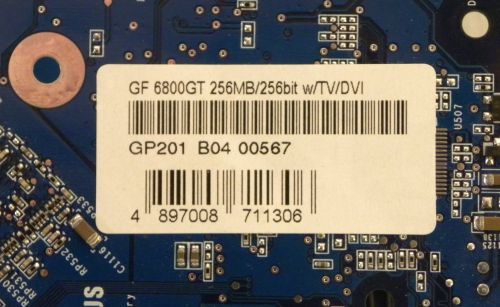This is an useless test, but I had the oppotunity to quickly test the latest R270.51 with a GeForce 6 card (AGP) in order to verify if latest NV drivers still support older cards:

This driver package supports GeForce 6, 7, 8, 9, 100, 200, 300, 400, and 500-series desktop GPUs as well as ION desktop GPUs.

I tested R270.51 with a GeForce 6800 GT under WinXP 32-bit: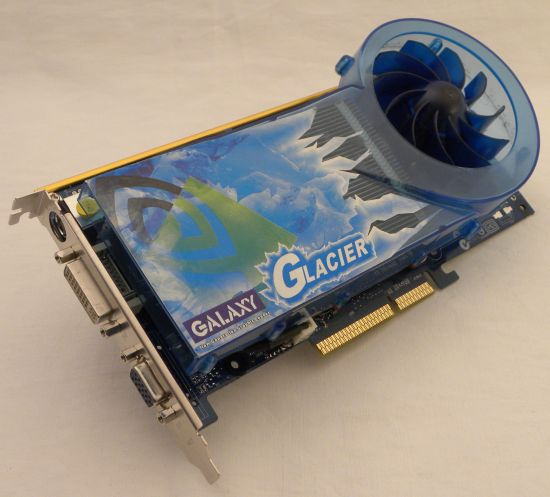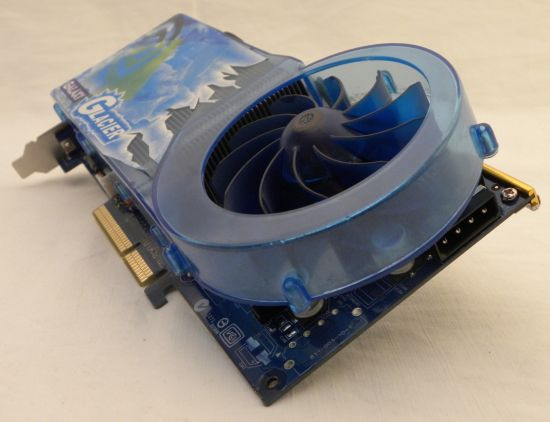I used the following tools: GPU Caps Viewer 1.11.1, GPU Shark 0.4.4 and FurMark (1.9.0 and 1.8.2).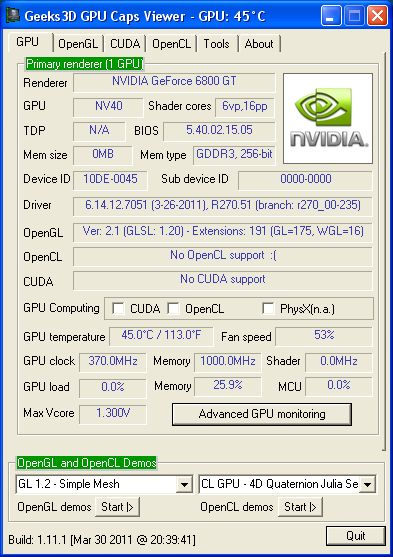GPU Caps Viewer 1.11.1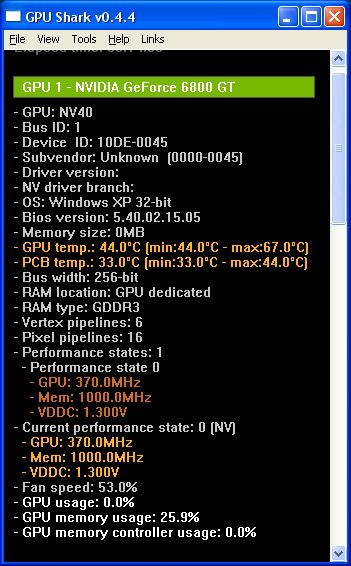GPU Shark 0.4.4

I successfully ran FurMark and here are the scores (1920×1080, fullscreen, 60 sec, no AA):
FurMark 1.8.2: 363 points (6 FPS)
FurMark 1.9.0: 81 points (1 FPS)

The verdict: the latest NVIDIA graphics driver (270 family) still support older GeForce 6. Keep in mind that GeForce 6 and GeForce 7 are non-unified architectures: there are specialized processors for vertex and pixels while GeForce 8 and up are based on unified architecture.

And to end up this post, here are the extensions exposed by R270.51 for a GeForce 6: 191 extensions (GL=175 and WGL=16)

• GL_ARB_color_buffer_float
• GL_ARB_copy_buffer
• GL_ARB_depth_clamp
• GL_ARB_depth_texture
• GL_ARB_draw_buffers
• GL_ARB_ES2_compatibility
• GL_ARB_explicit_attrib_location
• GL_ARB_fragment_program
• GL_ARB_framebuffer_object
• GL_ARB_half_float_pixel
• GL_ARB_half_float_vertex
• GL_ARB_imaging
• GL_ARB_map_buffer_range
• GL_ARB_multisample
• GL_ARB_multitexture
• GL_ARB_occlusion_query
• GL_ARB_occlusion_query2
• GL_ARB_pixel_buffer_object
• GL_ARB_point_parameters
• GL_ARB_point_sprite
• GL_ARB_provoking_vertex
• GL_ARB_robustness
• GL_ARB_sampler_objects
• GL_ARB_texture_border_clamp
• GL_ARB_texture_compression
• GL_ARB_texture_cube_map
• GL_ARB_texture_env_combine
• GL_ARB_texture_env_crossbar
• GL_ARB_texture_env_dot3
• GL_ARB_texture_float
• GL_ARB_texture_mirrored_repeat
• GL_ARB_texture_non_power_of_two
• GL_ARB_texture_rectangle
• GL_ARB_texture_rg
• GL_ARB_texture_swizzle
• GL_ARB_timer_query
• GL_ARB_transpose_matrix
• GL_ARB_vertex_array_bgra
• GL_ARB_vertex_array_object
• GL_ARB_vertex_buffer_object
• GL_ARB_vertex_program
• GL_ARB_window_pos
• GL_ATI_draw_buffers
• GL_ATI_texture_float
• GL_ATI_texture_mirror_once
• GL_S3_s3tc
• GL_EXT_abgr
• GL_EXT_bgra
• GL_EXT_blend_color
• GL_EXT_blend_equation_separate
• GL_EXT_blend_func_separate
• GL_EXT_blend_minmax
• GL_EXT_blend_subtract
• GL_EXT_compiled_vertex_array
• GL_EXT_depth_bounds_test
• GL_EXT_direct_state_access
• GL_EXT_draw_range_elements
• GL_EXT_fog_coord
• GL_EXT_framebuffer_blit
• GL_EXT_framebuffer_multisample
• GL_EXT_framebuffer_object
• GL_EXT_gpu_program_parameters
• GL_EXT_multi_draw_arrays
• GL_EXT_packed_depth_stencil
• GL_EXT_packed_pixels
• GL_EXT_pixel_buffer_object
• GL_EXT_point_parameters
• GL_EXT_provoking_vertex
• GL_EXT_rescale_normal
• GL_EXT_secondary_color
• GL_EXT_separate_specular_color
• GL_EXT_stencil_two_side
• GL_EXT_stencil_wrap
• GL_EXT_texture3D
• GL_EXT_texture_compression_dxt1
• GL_EXT_texture_compression_s3tc
• GL_EXT_texture_cube_map
• GL_EXT_texture_edge_clamp
• GL_EXT_texture_env_combine
• GL_EXT_texture_env_dot3
• GL_EXT_texture_filter_anisotropic
• GL_EXT_texture_format_BGRA8888
• GL_EXT_texture_lod
• GL_EXT_texture_lod_bias
• GL_EXT_texture_mirror_clamp
• GL_EXT_texture_object
• GL_EXT_texture_sRGB
• GL_EXT_texture_swizzle
• GL_EXT_timer_query
• GL_EXT_vertex_array
• GL_EXT_vertex_array_bgra
• GL_IBM_rasterpos_clip
• GL_IBM_texture_mirrored_repeat
• GL_KTX_buffer_region
• GL_NV_alpha_test
• GL_NV_blend_minmax
• GL_NV_blend_square
• GL_NV_complex_primitives
• GL_NV_copy_depth_to_color
• GL_NV_depth_clamp
• GL_NV_fbo_color_attachments
• GL_NV_fence
• GL_NV_float_buffer
• GL_NV_fog_distance
• GL_NV_fragdepth
• GL_NV_fragment_program
• GL_NV_fragment_program_option
• GL_NV_fragment_program2
• GL_NV_framebuffer_multisample_coverage
• GL_NV_half_float
• GL_NV_light_max_exponent
• GL_NV_multisample_filter_hint
• GL_NV_occlusion_query
• GL_NV_packed_depth_stencil
• GL_NV_pixel_data_range
• GL_NV_point_sprite
• GL_NV_primitive_restart
• GL_NV_register_combiners
• GL_NV_register_combiners2
• GL_NV_texgen_reflection
• GL_NV_texture_barrier
• GL_NV_texture_compression_vtc
• GL_NV_texture_env_combine4
• GL_NV_texture_expand_normal
• GL_NV_texture_lod_clamp
• GL_NV_texture_rectangle
• GL_NV_vertex_array_range
• GL_NV_vertex_array_range2
• GL_NV_vertex_program
• GL_NV_vertex_program1_1
• GL_NV_vertex_program2
• GL_NV_vertex_program2_option
• GL_NV_vertex_program3
• GL_NVX_conditional_render
• GL_OES_depth24
• GL_OES_depth32
• GL_OES_depth_texture
• GL_OES_element_index_uint
• GL_OES_fbo_render_mipmap
• GL_OES_get_program_binary
• GL_OES_mapbuffer
• GL_OES_packed_depth_stencil
• GL_OES_rgb8_rgba8
• GL_OES_standard_derivatives
• GL_OES_texture_3D
• GL_OES_texture_float
• GL_OES_texture_float_linear
• GL_OES_texture_half_float
• GL_OES_texture_half_float_linear
• GL_OES_texture_npot
• GL_OES_vertex_array_object
• GL_OES_vertex_half_float
• GL_SGIS_generate_mipmap
• GL_SGIS_texture_lod
• GL_SGIX_depth_texture
• GL_SUN_slice_accum
• GL_WIN_swap_hint
• WGL_EXT_swap_control
• WGL_ARB_buffer_region
• WGL_ARB_create_context
• WGL_ARB_create_context_profile
• WGL_ARB_create_context_robustness
• WGL_ARB_extensions_string
• WGL_ARB_multisample
• WGL_ARB_pbuffer
• WGL_ARB_pixel_format
• WGL_ARB_pixel_format_float
• WGL_ARB_render_texture
• WGL_ATI_pixel_format_float
• WGL_EXT_extensions_string
• WGL_NV_float_buffer
• WGL_NV_render_depth_texture
• WGL_NV_render_texture_rectangle

## 5 thoughts on “(Test) R270.51 and GeForce 6: Still supported?”

1.Psolord

Ah the 6800GT. Could play Half Life 2 at 1600X1200@60fps in 2004 and the retarded game consoles have taken resolutions back to 1280X720 and the framerate to 30fps! Ok different load but still, Jesus Christ!

2.Arun

This is one Reason i trust Nvidia .. They still support there products even it is 5 years old ..

3.Promilus

@Arun – tell it to those who receive bsod or 4bit color when updating forceware on eg. GF7600GS. One thing for which it is worth a try is:
GL_ARB_ES2_compatibility

4.Korvin77

Thanks for testing 😉

“Keep in mind that GeForce 6 and GeForce 7 are non-unified architectures: there are specialized processors for vertex and pixels while GeForce 8 and up are based on unified architecture.”

LOL it was not so long ago, we remember 😛

5.Squall Leonhart

They really should dump the AGP support, because of it we aren’t getting optimal instruction optimisations.